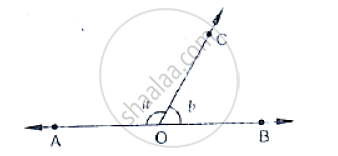# In the Below Fig, ∠Aoc and ∠Boc Form a Linear Pair. If a − 2b = 30°, Find a and B. - Mathematics

In the below Fig, ∠AOC and ∠BOC form a linear pair. if a − 2b = 30°, find a and b.#### Solution

Given that,

∠AOC and  ∠BOC form a linear pair

If a - 2b = 30°

∠AOC = a°, ∠BOC = b°

∴a + b = 180°              ..........(i)

Given a - 2b = 30°                ............(ii)

By subtracting (i) and (ii)

a + b - a + 2b = 180° - 30°

⇒ 3b = 150°

⇒ b = (150°)/3

⇒ b = 50°

Hence a - 2b = 30°

a - 2 (50)° = 30°                                    [∴   b = 50°]

a = 30° +100°

a = 130°

∴ a = 130°, b = 50°

Concept: Pairs of Angles
Is there an error in this question or solution?
Chapter 10: Lines and Angles - Exercise 10.2 [Page 15]

#### APPEARS IN

RD Sharma Mathematics for Class 9
Chapter 10 Lines and Angles
Exercise 10.2 | Q 5 | Page 15

Share